# How to Convert Letter to Number or Vice Versa in Excel?

Sometimes in Excel, for encrypting the data, you have needed to convert letters to numbers based on their position in the alphabet series. For example, a = 1, b = 2, z = 26, and so on. Read this tutorial to learn how we can convert a letter to a number or a number to a letter based on their position in Excel. We can complete this process using the formulas supported in Excel. Mostly, this process is used for encryption and decryption of the data. Both encryption and decryption are used to provide security for data.

## Converting Letters to Numbers in Excel

Here, we will first use the column formula to get the first result, then use the autofill handle to get all the results. Let's go over a simple procedure for converting a letter to a number in Excel using formulas.

### Step 1

Let us consider any Excel sheet that contains a list of letters as similar to the below image.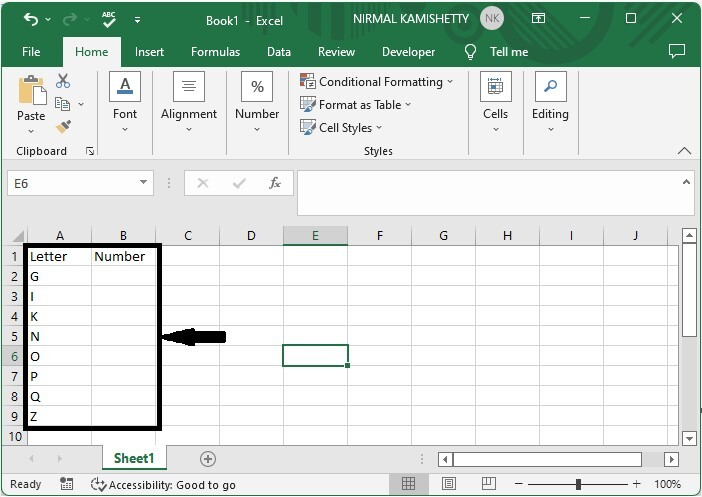Then, in our case, cell B1, click on an empty cell and enter the formula as follows −

=COLUMN(INDIRECT(A2&1)) and click Enter to get our first result, as shown in the below image.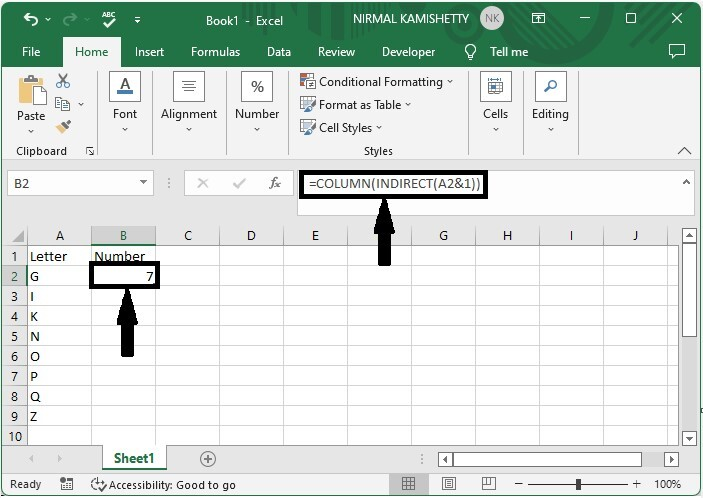### Step 2

To get all the results drag down from the first result and our final result will be similar to below image.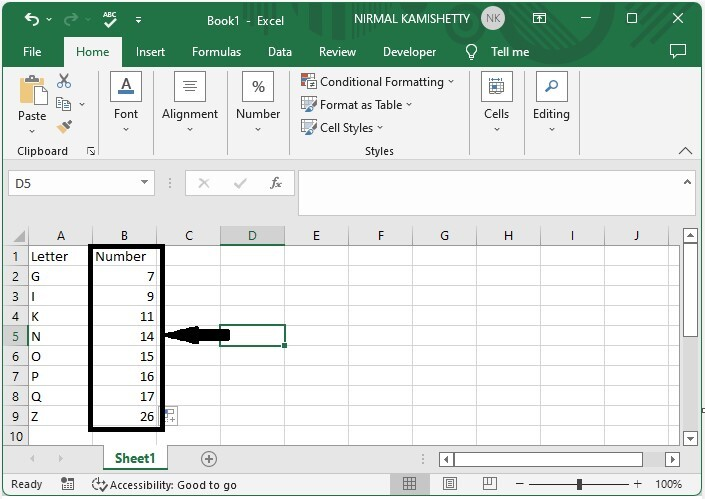## Converting Numbers to Letters in Excel

Here we will use the MID formula to get any one of the results, then use the auto-fill handle to get all the results. Let's go over a simple procedure for converting numbers to letters in Excel using formulas.

### Step 1

Let us consider an Excel sheet that contains a list of numbers similar to the one below. The numbers should always be less than 26.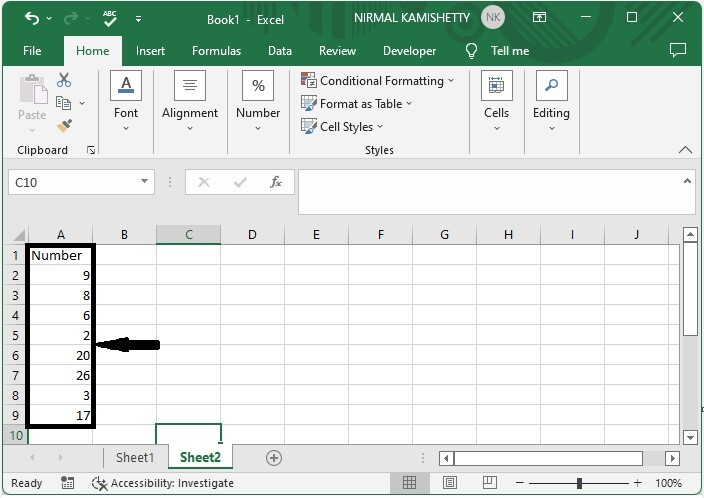Now click on the empty cell and enter the formula as

=MID("ABCDEFGHIJKLMNOPQRSTUVWXYZ",A2,1) and click enter to get our first result.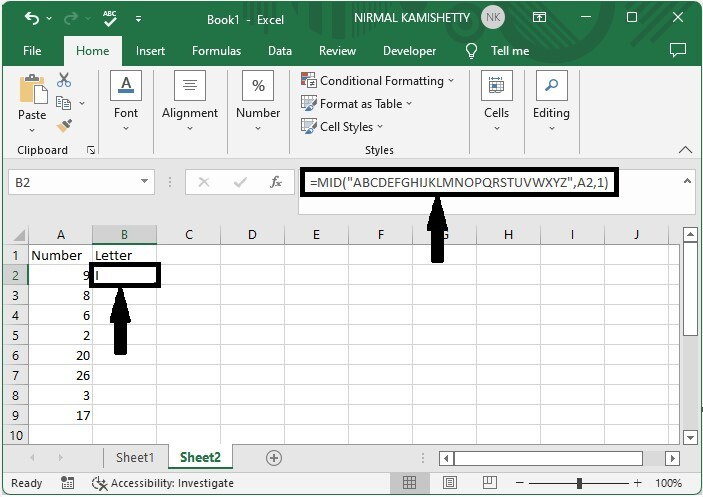### Step 2

Drag down from the first result using the auto-fill handle to get all the results, and our final result will be similar to the below image.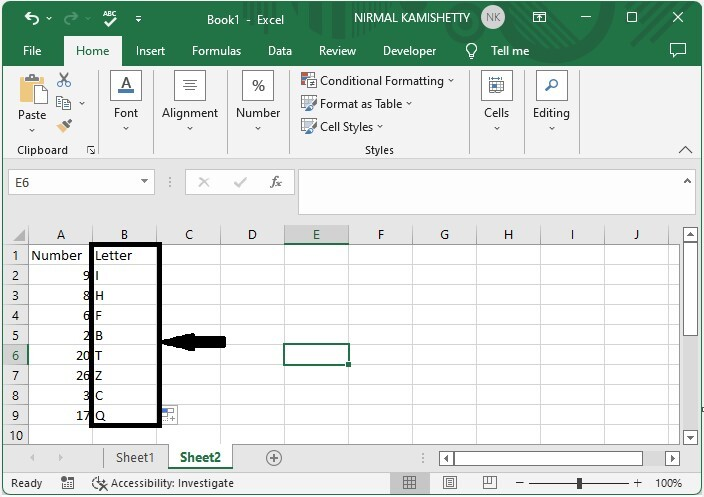## Conclusion

In this tutorial, we used a simple example to demonstrate how you can convert a letter to a number or vice versa in Excel.